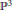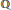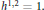Hostname: page-component-546b4f848f-fhndm Total loading time: 0 Render date: 2023-06-01T21:50:31.994Z Has data issue: false Feature Flags: { "useRatesEcommerce": true } hasContentIssue false

# Geometry and Arithmetic of Certain Double Octic Calabi–Yau Manifolds

Published online by Cambridge University Press:  20 November 2018

## Abstract

HTML view is not available for this content. However, as you have access to this content, a full PDF is available via the ‘Save PDF’ action button.

We study Calabi–Yau manifolds constructed as double coverings of${{\mathbb{P}}^{3}}$ branched along an octic surface. We give a list of 87 examples corresponding to arrangements of eight planes defined over$\mathbb{Q}$. The Hodge numbers are computed for all examples. There are 10 rigid Calabi–Yau manifolds and 14 families with${{h}^{1,2}}\,=\,1.$ The modularity conjecture is verified for all the rigid examples.

## Keywords

Type
Research Article
Information
Canadian Mathematical Bulletin , 01 June 2005 , pp. 180 - 194

## References

 Cynk, S., Double coverings of octic arrangements with isolated singularities. Adv. Theor. Math. Phys. 3(1999), 217225.CrossRefGoogle Scholar
 Cynk, S., Cohomologies of a double covering of a non–singular algebraic 3-fold. Math. Z. 240 (2002), 731743.CrossRefGoogle Scholar
 Cynk, S. and van Straten, D., Infinitesimal deformations of smooth algebraic varieties. To appear in Math. Nachrichten. Preprint (2003), AG/0303329.Google Scholar
 Cynk, S. and Szemberg, T., Double covers and Calabi–Yau varieties. Banach Center Publ. 44, Polish Acad. Sci., Warsaw, 1998, pp. 93101.Google Scholar
 Dieulefait, L. and Manoharmayum, J., Modularity of rigid Calabi-Yau threefolds over . In: Calabi-Yau varieties and mirror symmetry. Proceedings of the Workshop on Arithmetic, Geometry and Physics around Calabi-Yau Varieties andMirror Symmetry (Toronto, 2001). Yui, Noriko and Lewis, James D., eds. Fields Institute Communications, 38, American Mathematical Society, Providence, RI, 2003, pp. 159166.Google Scholar
 Esnault, H. and Viehweg, E., Lectures on vanishing theorems. DMV Seminar 20, Birkhäuser Verlag, Basel, 1992.CrossRefGoogle Scholar
 Greuel, G.-M., Pfister, G., and Schönemann, H.. Singular 2.0. A Computer Algebra System for Polynomial Computations. Centre for Computer Algebra, University of Kaiserslautern (2001). http://www.singular.uni-kl.de.Google Scholar
 Hulek, K., Spandaw, J., van Geemen, B., and van Straten, D., The modularity of the Barth–Nieto quintic and its relatives. Adv. Geom. 1(2001), 263289.CrossRefGoogle Scholar
 Meyer, C., A Dictionary of Modular Threefolds. Thesis, Mainz, 2005.Google Scholar
 Saito, M. and Yui, N., The modularity conjecture for rigid Calabi–Yau threefolds over Q. J. Math. Kyoto Univ. 41(2001), 403419.CrossRefGoogle Scholar
 Stein, W. A., Modular forms database. http://modular.fas.harvard.edu/.Google Scholar
 Szendröi, B., Calabi–Yau threefolds with a curve of singularities and counterexamples to the Torelli problem. Internat. J. Math. 11(2000), 449459.CrossRefGoogle Scholar
 Szendröi, B., Calabi–Yau threefolds with a curve of singularities and counterexamples to the Torelli problem. II. Math. Proc. Cambridge Philos. Soc. 129(2000), 193204.CrossRefGoogle Scholar
 Verrill, H. A., The L-series of certain rigid Calabi–Yau threefolds. J. Number Theory 81(2000), 310334.CrossRefGoogle Scholar
 Wilson, P. M. H., The Kähler cone on Calabi–Yau threefolds. Invent. Math. 107(1992), 561583.CrossRefGoogle Scholar